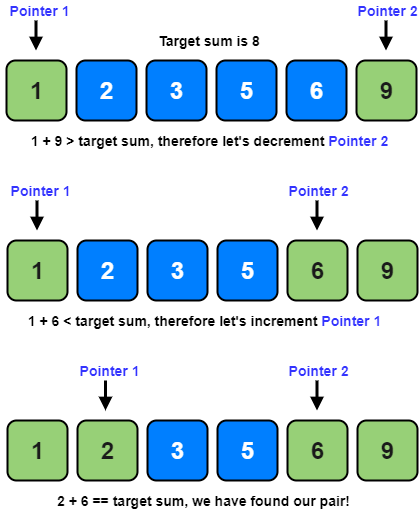# Coding Patterns: Two Pointers

In Coding Patterns series, we will try to recognize common patterns underlying behind each algorithm question, using real examples from Leetcode.

Previous post was about Sliding Window pattern and today, we will introduce Two Pointers pattern which is very useful to solve problems with sorted arrays (or Linked Lists) which involve a set of pair elements, or a triplet or even a subarray.

## Problem: Pair with Target Sum

LeetCode 1 - Two Sum [easy]

Given an array of integers, return indices of the two numbers such that they add up to a specific target.

You may assume that each input would have exactly one solution, and you may not use the same element twice.

Example:

``````Given nums = [2, 7, 11, 15], target = 9,

Because nums + nums = 2 + 7 = 9,
return [0, 1].
``````

### Two Pointers Solution

With using the Two Pointers pattern, and Pointer 1 pointing to the beginning of the array and Pointer 2 pointing to the end of the array, we will check if the numbers pointed by the pointers add up to the target sum. If they do, we have found our pair. If not, we should do one of these things:

• If the sum is bigger than the target sum, this means that we need a smaller sum so, we are going to decrement the Pointer 2 (end-pointer).
• If the sum is smaller than the target sum, this means that we need a bigger sum so, we are going to increment the Pointer 1 (start-pointer).Please note that, this solution only works if we are working with sorted arrays!

``````def twoSum(self, nums: List[int], target: int) -> List[int]:
left, right = 0, len(nums) - 1
while left < right:
current_sum = nums[left] + nums[right]
if current_sum == target:
return [left, right]

if target > current_sum:
left += 1  # we need a pair with a bigger sum
else:
right -= 1  # we need a pair with a smaller sum
return [-1, -1]
``````

Time Complexity: O(N) where `N` is the number of elements in the input array (`nums`).

Space Complexity: O(1), algorithm runs in constant space.

### An Alternative Solution

Instead of using the Two Pointers Solution, we can use a HashTable to solve the problem.

We are searching the array for 2 items, `x` and `y` where `x + y = target`. This means that, during our iteration when we are at number `x`, we are looking for a `y` (which is equivalent to `target - x`, basic maths!).

If we found a `target - x` value in HashTable, we found our pair! If not, we will insert `x` into the HashTable, so that we can search for it later.

``````def twoSum(self, nums: List[int], target: int) -> List[int]:
num_map = {}  # to store numbers and their indices
for i, num in enumerate(nums):
if target - num in num_map:
return [num_map[target - num], i]
else:
num_map[nums[i]] = i
return [-1, -1]
``````

Time Complexity: O(N) where `N` is the number of elements in the input array (`nums`).

Space Complexity: O(N), in the worst case, we will be pushing N numbers to HashMap.

## How to identify?

So we want to be able to identify the problems that Two Pointers pattern works.

• The problem involve sorted arrays (or Linked Lists), a set of pair elements, or a triplet or even a subarray.
• There is a target value to match or duplicates to be removed.

Most of these type of problems can be solved in O(N) time complexity and O(1) or O(N) space complexity.

Tags:

Categories:

Updated: